maths > wholenumbers

Multiplication : Simplified Procedure for Large Numbers

what you'll learn...

overview

The multiplication in first principles was explained as repetition of multiplicand, the multiplier number of times.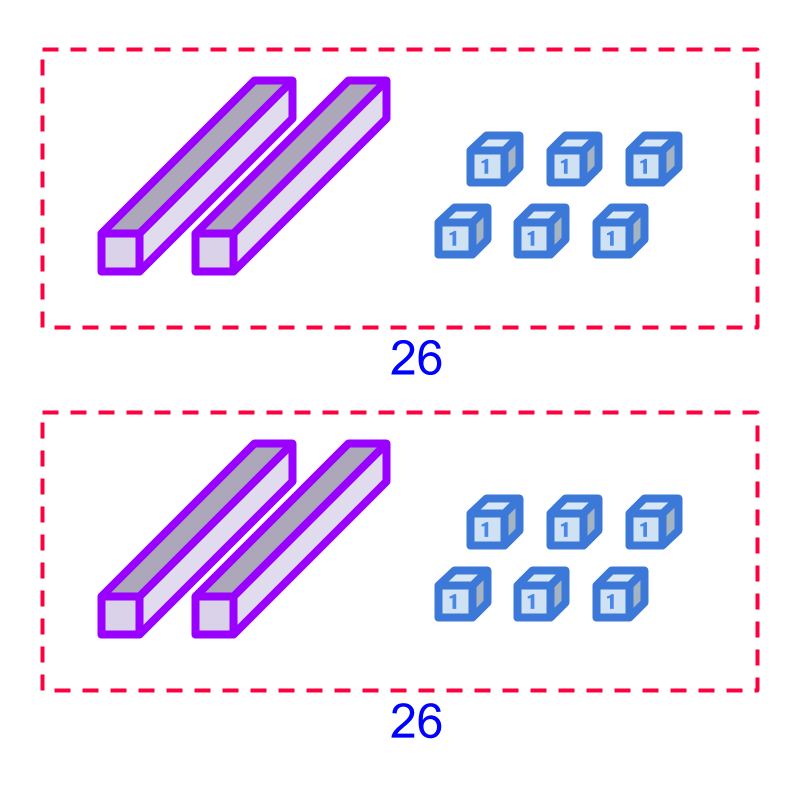This method is revised for 2-digit numbers and established that the regrouping of units, tens, etc. is required.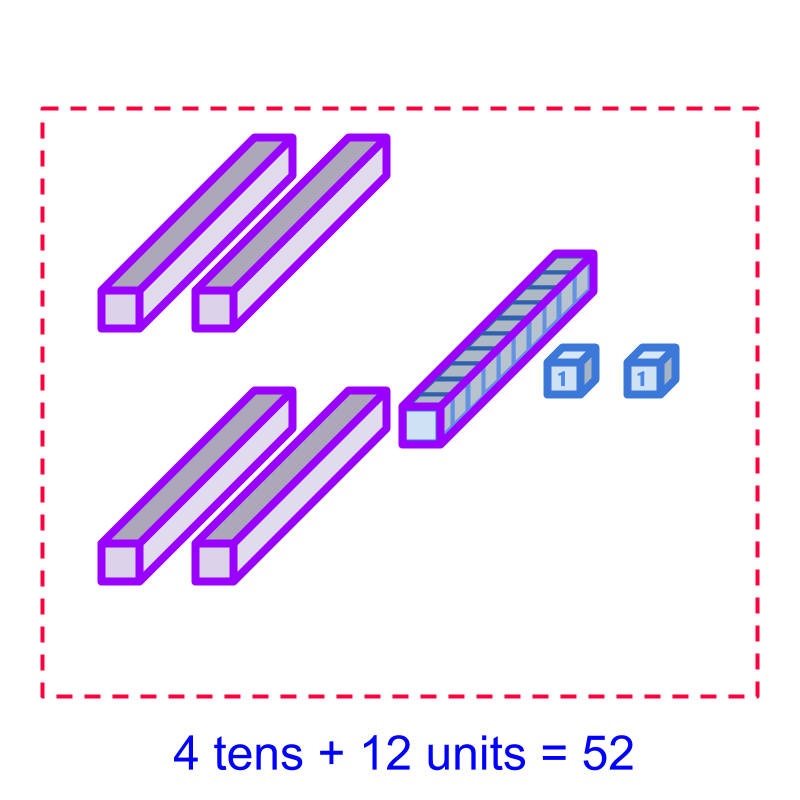This establishes the simplified procedure : multiplication by place-value with regrouping.

regrouping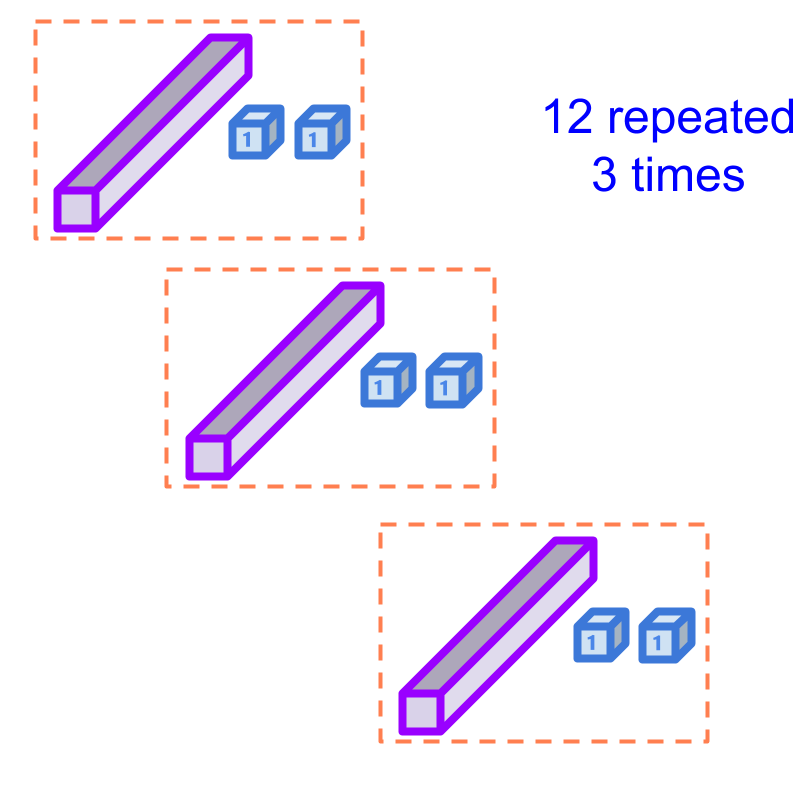Consider multiplication of 12$12$ and 3$3$.

This is performed as, combine 12$12$ repeatedly 3$3$ times and count the combined quantity.

Considering the multiplication 12×3$12 \times 3$.

Combining 12$12$ repeatedly 3$3$ times is shown in the figure. There are $3$ tens and $6$ units which together form value $36$.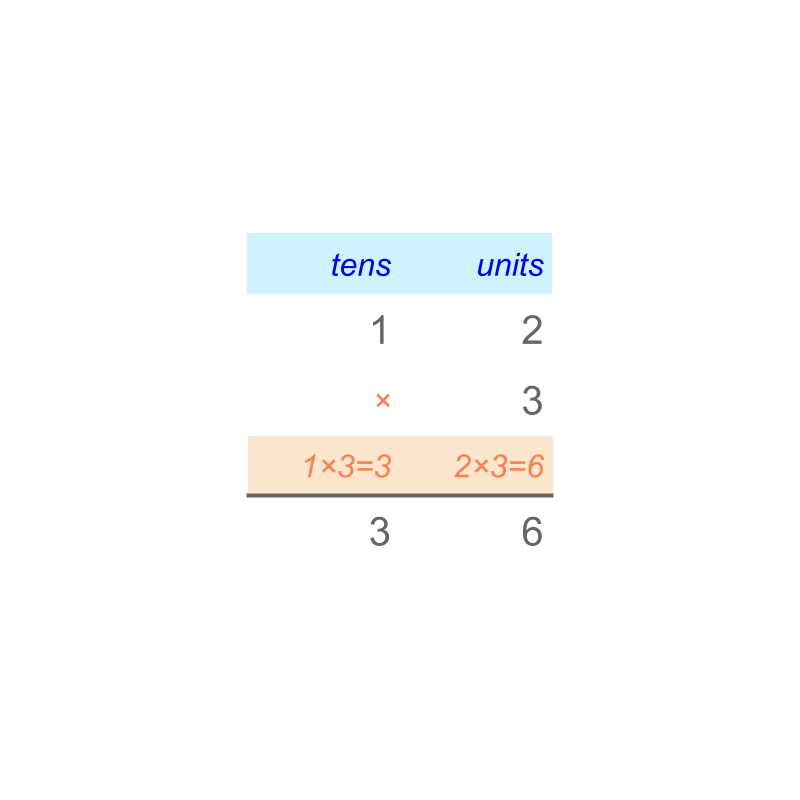Considering the multiplication $12 \times 3$. The number $12$ is given in the place value format in the figure.

The repeated addition is visualized and the multiplication is performed as illustrated in the figure.

The units place is multiplied as $2 \times 3 = 6$ and the tens place is multiplied as $1 \times 3 = 3$. The result of the multiplication is $36$.Consider the multiplication $26 \times 2$. That is, $26$ is repeated twice as shown in the figure. Counting the two quantities together, there are $4$ tens and $12$ units. The combined value is $52$.Considering the multiplication $26 \times 2$. That is, $26$ is repeated twice and combined as shown in the figure.
Note that $10$ units are regrouped together to form the $1$ ten. So the result of the multiplication is $52$.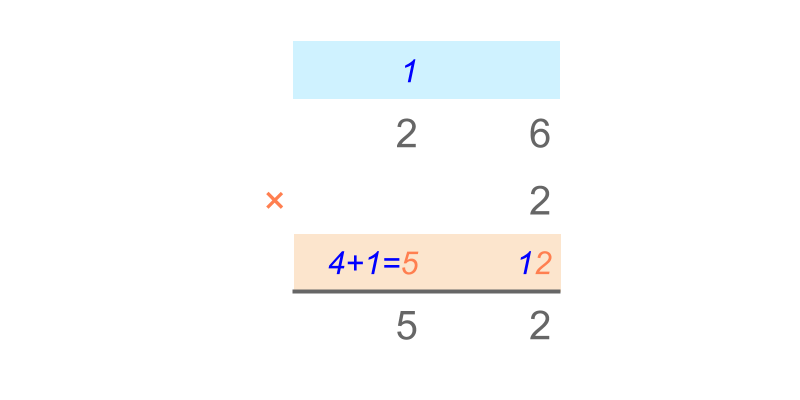Considering the multiplication of $26 \times 2$. The number $26$ is given in the place value format in the figure.

A simplified procedure Multiplication by Place-value with regrouping is given in the figure.

In the units place, $6$ and $2$ are multiplied to $12$. The $10$ unit is converted to $1$ ten and moved to the tens position. This regrouping $10$ of lower place-value to $1$ of the immediate higher place-value is carry over. The remaining units $2$ is retained.

In the tens place, $2$ and $2$ are multiplied to $4$ and the carry over is added to that. So, the tens place of result is $4 + 1 = 5$.

The result is $52$.

simplify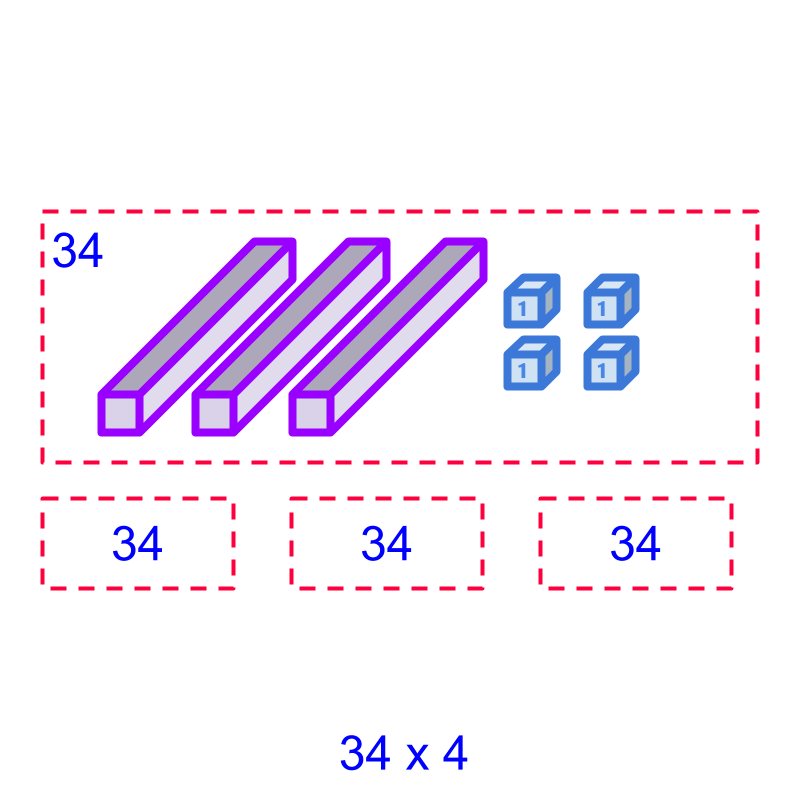Consider the multiplication: $34 \times 4$. One $34$ is shown in the figure.

To multiply, the quantity $34$ can be repeated $4$ times or the simplified procedure "multiplication by place-value with regrouping" can be used.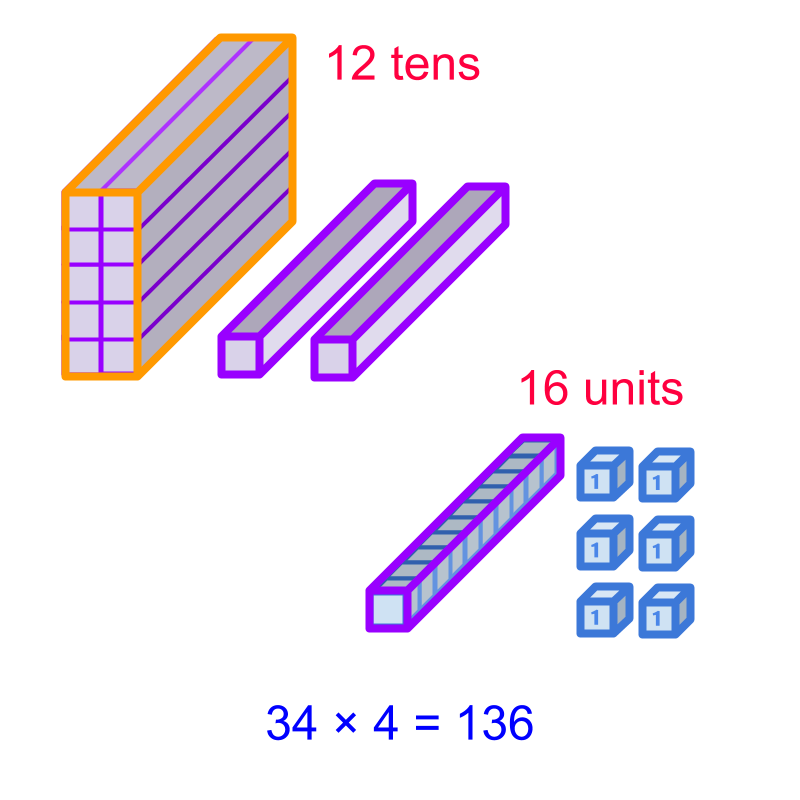Considering the multiplication: $34 \times 4$. The value $34$ is repeated $4$ times and combined as shown in the figure. The combined quantity makes $12$ tens and $16$ units.

The $10$ tens are regrouped into $1$ hundred. The $10$ units are regrouped into $1$ ten. Then the number of hundreds, tens, and units are counted to the result $136$.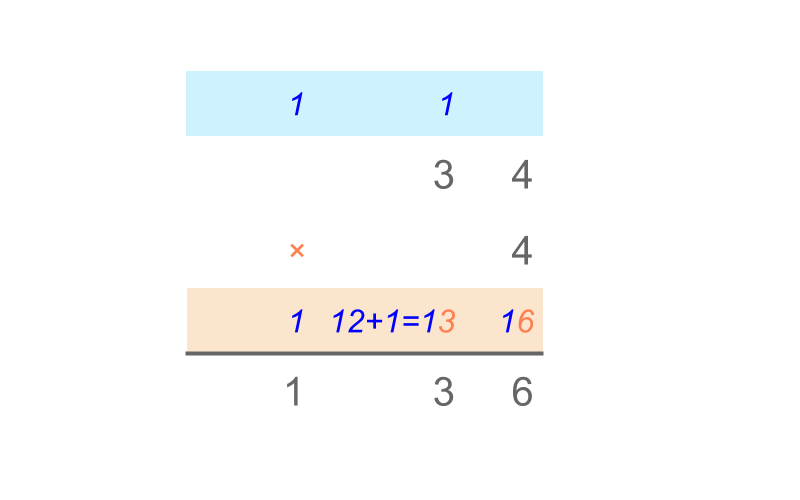Considering the multiplication: $34 \times 4$. The numbers are given in the place value in the figure.

A simplified procedure, Multiplication by Place-value is given in the figure.

The digit in the units place is multiplied by the multiplier. The tens formed is carried over to the tens position,
Then the digit in the tens place is multiplied by the multiplier. The hundreds formed is carried over to the hundreds position.

The result of the multiplication is $136$.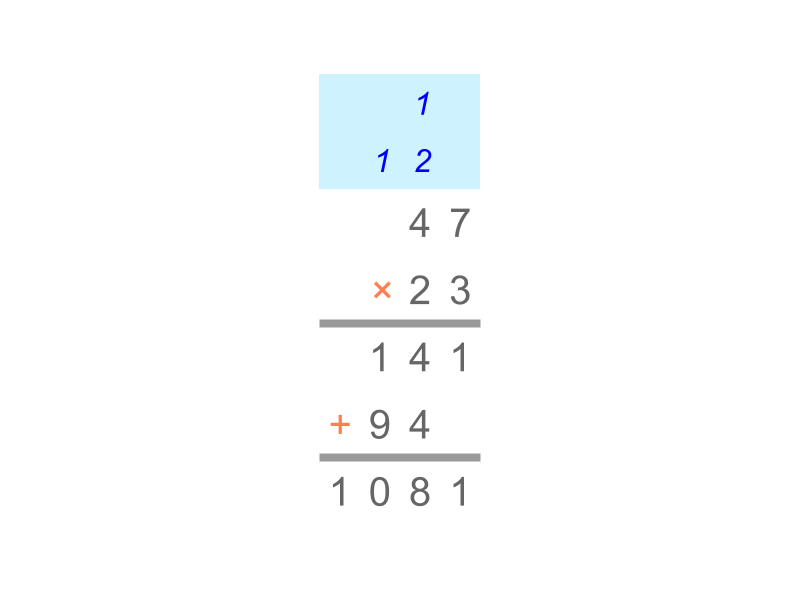Consider the multiplication of $47$ and $23$. The numbers are shown in the place value format in the figure.

The multiplication in place value is illustrated. The product is $1081$.

Multiplication by Place-value with Regrouping - Simplified Procedure : Two numbers are multiplied as illustrated in the figure.
Note: The procedure combines $10$ units into $1$ ten as carry over and so on for higher place-values.

examples

What is $284 \times 20$?
The answer is "$5680$"

What is $11 \times 123$?
The answer is "$1353$"

summary

Multiplication by Place-value - Simplified Procedure : Two numbers are multiplied as illustrated in the figure.Note: The procedure combines $10$ units into $1$ ten as carry over and so on for higher place-values.

Outline